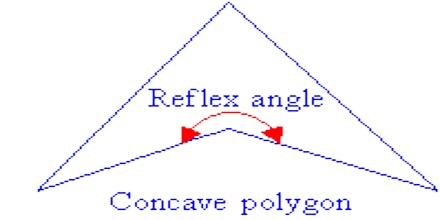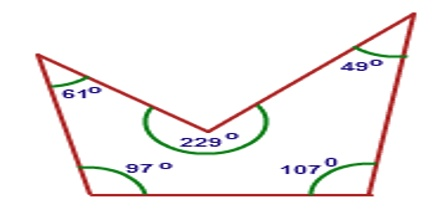Concave polygon is a polygon that has one or more interior angles greater than 180°. A polygon with at least one interior angle greater than 1800 is a concave polygon. Concave polygon must have at least four sides. A triangle can never be a concave polygon, because it has three angles and the sum of all three angles cannot be greater than 180°. Therefore, one of the angle can never be a reflex angle which must be satisfied for a polygon to be a concave polygon.Properties of Concave Polygon

A concave polygon has the following properties –

• It has at least one interior angle greater than 1800 and less than 3600. We can say that a concave polygon has at least one angle a reflex angle.
• It has at least one vertex pointing inwards which gives it a concave shape.
• At least one pair of sides joining a vertex goes outside the vertex.
• There can be one more than one diagonals which lie outside the boundary of the polygon.
• A line segment which is drawn crossing the concave polygon, intersects its boundary more than two times.Overview

A line drawn through a concave polygon, depending on exactly where you draw it, can intersect the polygon in more than two places. In the figure on the right, the line cuts the polygon in 4 places. Some of the diagonals of a concave polygon will lie outside the polygon.

The area of a concave polygon can be found by treating it as any other irregular polygon.